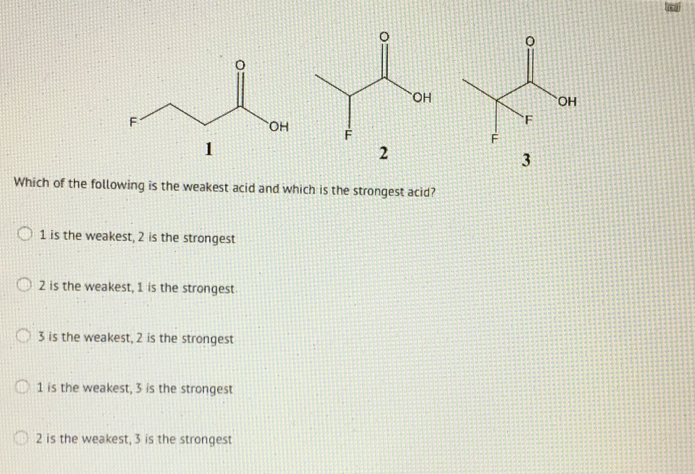# Problem: Which of the following is the weakest acid and which is the strongest acid? a. 1 is the weakest, 2 is the strongest b. 2 is the weakest, 1 is the strongest c. 3 is the weakest, 2 is the strongest d. 1 is the weakest, 3 is the strongest e. 2 is the weakest, 3 is the strongest

###### FREE Expert Solution
89% (32 ratings)###### Problem Details

Which of the following is the weakest acid and which is the strongest acid?

a. 1 is the weakest, 2 is the strongest

b. 2 is the weakest, 1 is the strongest

c. 3 is the weakest, 2 is the strongest

d. 1 is the weakest, 3 is the strongest

e. 2 is the weakest, 3 is the strongest